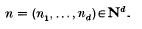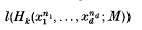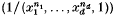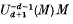Hostname: page-component-7d8f8d645b-xmxxh Total loading time: 0 Render date: 2023-05-29T09:14:19.261Z Has data issue: false Feature Flags: { "useRatesEcommerce": true } hasContentIssue false

# On the lengths of Koszul homology modules and generalized fractions

Published online by Cambridge University Press:  24 October 2008

## Extract

Throughout this paper, let A be a Noetherian local ring with maximal ideal m and M a finitely generated A-module with d = dimAM ≥ 1. Denote by N the set of all positive integers.

Let x = (x1, …, xd) be a system of parameters (s.o.p) for M and letWe consider the following two problems: (i) When is the length of Koszul homologya polynomial in n for all k = 0, …, d and n1; …, nd sufficiently large (n ≫ 0)?

(ii) Is the length of the generalized fractionina polynomial in n for n ≫ 0?

Type
Research Article

## Access options

Get access to the full version of this content by using one of the access options below. (Log in options will check for institutional or personal access. Content may require purchase if you do not have access.)

## References

#### REFERENCES

Auslander, M. and Buchsbaum, D. A.. Codimension and multiplicity. Ann. Math. 68 (1958), 625657.CrossRefGoogle Scholar
Cuong, N. T.. On the length of the powers of systems of parameters in local ring. Nagoya Math. J. 120 (1990), 7788.CrossRefGoogle Scholar
Cuong, N. T.. On the dimension of the non-Cohen-Macaulay locus of local ring admitting dualizing complexes. Math. Proc. Cambridge Phil. Soc. (2) 109 (1991), 479488.CrossRefGoogle Scholar
Cuong, N. T.. On the least degree of polynomials bounding above the differences between lengths and multiplicities of certain systems of parameters in local rings. Nagoya Math. J. 125 (1992), 105114.CrossRefGoogle Scholar
Cuong, N. T.. The theory of polynomial types and p-standard ideals in local rings and applications, preprint.Google Scholar
Cuong, N. T., Schenzel, P. and Trung, N. V.. Verallgemeinerte Cohen-Macaulay Moduln. Math. Nach. 85 (1978), 5775.Google Scholar
Garcla Roig, J- I.. On polynomial bounds for the Koszul homology of certain multiplicity systems. J. London Math. Soc. (2) 34 (1986), 411416.CrossRefGoogle Scholar
Goto, S. and Yamagishi, K.. The theory of unconditional strong d-sequences and modules of finite local cohomology, preprint.Google Scholar
Kaplansky, I.. Commutative rings (Allyn and Bacon, 1970).Google Scholar
Kirby, D.. Artinian modules and Hilbert polynomials. Quart. J. Math. Oxford (2) 24 (1973), 4757.CrossRefGoogle Scholar
MacDonald, I. G.. Secondary representation of modules over a commutative ring. Sympos. Math. 11 (1973), 2343.Google Scholar
Matsumura, H.. Commutative algebra. Second Edition (Benjamin, 1980).Google Scholar
Schenzel, P.. Cohomological annihilators. Math. Proc. Cambridge Phil. Soc. 91 (1982), 345350.CrossRefGoogle Scholar
Sharp, R. Y. and Hamieh, M. A.. Lengths of certain generalized fractions. J. Pure Appl. Algebra 38 (1985), 323336.CrossRefGoogle Scholar
Sharp, R. Y. and Zakeri, H.. Modules of generalized fractions. Mathematika 29 (1982), 3241.CrossRefGoogle Scholar
Sharp, R. Y. and Zakeri, H.. Local cohomology and modules of generalized fractions. Mathematika 29 (1982), 296306.CrossRefGoogle Scholar
Sharp, R. Y. and Zakeri, H.. Generalized fractions and the monomial conjecture. J. Algebra 92 (1985), 380388.CrossRefGoogle Scholar
Trung, N. V.. Toward a theory of generalized Cohen–Macaulay modules. Nagoya Math. J. 102 (1986), 149.CrossRefGoogle Scholar